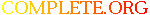Complete.Org: Mailing Lists: Archives: freeciv-dev: January 2005: [Freeciv-Dev] (PR#12022) duplicate free when destroying or taking over a# [Freeciv-Dev] (PR#12022) duplicate free when destroying or taking over a

[Top] [All Lists]

 To: bh@xxxxxxxxxxxxxxxxxxx Subject: [Freeciv-Dev] (PR#12022) duplicate free when destroying or taking over a city From: "Jason Short" Date: Fri, 28 Jan 2005 00:02:02 -0800 Reply-to: bugs@xxxxxxxxxxx

```<URL: http://bugs.freeciv.org/Ticket/Display.html?id=12022 >

There are two bugs here.

==4246== Invalid read of size 4
==4246==    at 0x8063200: reality_check_city (citytools.c:1650)
==4246==    by 0x8064084: remove_city (citytools.c:1119)
==4246==    by 0x8064F68: handle_unit_enter_city (citytools.c:1199)
==4246==    by 0x805B6A9: handle_unit_move_consequences (unittools.c:2619)
==4246==    by 0x805BF6A: move_unit (unittools.c:2883)
==4246==    by 0x809618B: handle_unit_move_request (unithand.c:1152)
==4246==    by 0x807025C: server_handle_packet (hand_gen.c:143)
==4246==    by 0x804ECC7: handle_packet_input (srv_main.c:994)
==4246==    by 0x809053E: sniff_packets (sernet.c:627)
==4246==    by 0x804F7CB: main_loop (srv_main.c:1557)
==4246==    by 0x8050993: srv_main (srv_main.c:1892)
==4246==    by 0x804A4B4: main (civserver.c:209)
==4246==  Address 0x1C78D618 is 0 bytes inside a block of size 44 free'd
==4246==    at 0x1B907460: free (vg_replace_malloc.c:153)
==4246==    by 0x8063216: reality_check_city (citytools.c:1651)
==4246==    by 0x8064084: remove_city (citytools.c:1119)
==4246==    by 0x8064F68: handle_unit_enter_city (citytools.c:1199)
==4246==    by 0x805B6A9: handle_unit_move_consequences (unittools.c:2619)
==4246==    by 0x805BF6A: move_unit (unittools.c:2883)
==4246==    by 0x809618B: handle_unit_move_request (unithand.c:1152)
==4246==    by 0x807025C: server_handle_packet (hand_gen.c:143)
==4246==    by 0x804ECC7: handle_packet_input (srv_main.c:994)
==4246==    by 0x809053E: sniff_packets (sernet.c:627)
==4246==    by 0x804F7CB: main_loop (srv_main.c:1557)
==4246==    by 0x8050993: srv_main (srv_main.c:1892)
==4246==    by 0x804A4B4: main (civserver.c:209)

==4246== Invalid free() / delete / delete[]
==4246==    at 0x1B907460: free (vg_replace_malloc.c:153)
==4246==    by 0x8063216: reality_check_city (citytools.c:1651)
==4246==    by 0x8064084: remove_city (citytools.c:1119)
==4246==    by 0x8064F68: handle_unit_enter_city (citytools.c:1199)
==4246==    by 0x805B6A9: handle_unit_move_consequences (unittools.c:2619)
==4246==    by 0x805BF6A: move_unit (unittools.c:2883)
==4246==    by 0x809618B: handle_unit_move_request (unithand.c:1152)
==4246==    by 0x807025C: server_handle_packet (hand_gen.c:143)
==4246==    by 0x804ECC7: handle_packet_input (srv_main.c:994)
==4246==    by 0x809053E: sniff_packets (sernet.c:627)
==4246==    by 0x804F7CB: main_loop (srv_main.c:1557)
==4246==    by 0x8050993: srv_main (srv_main.c:1892)
==4246==    by 0x804A4B4: main (civserver.c:209)
==4246==  Address 0x1C78D618 is 0 bytes inside a block of size 44 free'd
==4246==    at 0x1B907460: free (vg_replace_malloc.c:153)
==4246==    by 0x8063216: reality_check_city (citytools.c:1651)
==4246==    by 0x8064084: remove_city (citytools.c:1119)
==4246==    by 0x8064F68: handle_unit_enter_city (citytools.c:1199)
==4246==    by 0x805B6A9: handle_unit_move_consequences (unittools.c:2619)
==4246==    by 0x805BF6A: move_unit (unittools.c:2883)
==4246==    by 0x809618B: handle_unit_move_request (unithand.c:1152)
==4246==    by 0x807025C: server_handle_packet (hand_gen.c:143)
==4246==    by 0x804ECC7: handle_packet_input (srv_main.c:994)
==4246==    by 0x809053E: sniff_packets (sernet.c:627)
==4246==    by 0x804F7CB: main_loop (srv_main.c:1557)
==4246==    by 0x8050993: srv_main (srv_main.c:1892)
==4246==    by 0x804A4B4: main (civserver.c:209)

On an immediate glance this seems impossible.  I don't have time to
track it down at the moment so I'm just forwarding this on.

-jason

```